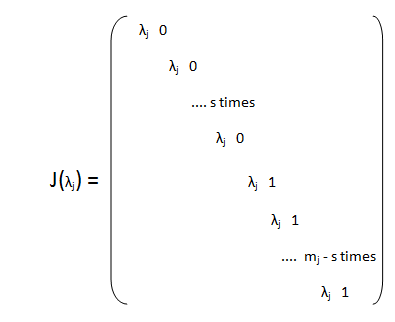# Canonical Jordan Form

### Basic Concepts and principles

Lets the endomorphism

$\begin{matrix} F:V\rightarrow V & \\ \,\,\,\,\,\,\,\,\,\,\,\,\,\,\,\,\,\,\,\,\,\,\,\,\,\,\,\,\,\,\,\,\,\,\,x \in V \rightarrow F(x) = Ax\in V \end{matrix}$

With V, vectorial K-n-dimensional space, n>1, and lets λj, with j = 1, 2, ...k, the roots of the characteristic polynomial of its associated Endomorphism with each of them with its multiplicity mj.

We have seen that for every Endomorphism there exists a nxn dimensional matrix such that

F(v) = Av

We are now at case of sum of each eigenspace dimensions generated by each eigenvalue is less than n, ie, the eigenvectors basis does not cover all the space V. In this case, the matrix is not diagonalizable, but it is possible to find a basis where the matrix V is expressed in a simpler form called Jordan form, and it is like

$$J=\begin{pmatrix} J_{1}& & & \\ & J_{2} & \\ & & ... & \\ & & & J_{n} \end{pmatrix}$$

Each one block J(λj) is called diagonal Jordan block and it is diagonal or has the form.

$$J_{j}=\begin{pmatrix} \lambda_{1}& 1 & & \\ & \lambda_{2} & 1 & \\ & & ... & 1 \\ & & & \lambda_{mj} \end{pmatrix}$$

### Theory

Lets F the Endomorphism

$\begin{matrix} F:V\rightarrow V & \\ \,\,\,\,\,\,\,\,\,\,\,\,\,\,\,\,\,\,\,\,\,\,\,\,\,\,\,\,\,\,\,\,\,\,\,x \in V \rightarrow F(x) = Ax\in V \end{matrix}$

And lets A the mxm-matrix associated with this Endomorphism.
Lets

$$P(x) = (x-\lambda_1)^{m_1}(x-\lambda_2)^{m_2} ... (x-\lambda_k)^{m_k}$$

The characteristic polynomial of A, with λj ∈K eigenvalues of A and the mj the corresponding multiplicity for each eigenvalue j, with j=1,2,...,k.

The following theorem tells us how V decomposes by the matrix A decomposed in the eigenspaces for each one eigenvalue

Theorem (Decomposition of subspaces corresponding to each eigenvalue)

Lets the eigenvalue λj and mj its multiplicity, then ∃p ∈ N such us

$E_1(\lambda_j)=ker(A-\lambda_jI)\subseteq E_2(\lambda_j)=ker(A-\lambda_jI)^2 \subseteq ... \subseteq E_1(\lambda_p)=ker(A-\lambda_jI)^p$

In addition, if $d_j=Dimension(E_p(\lambda_j))$ then it holds $d_1 \leq d_2 \leq ...\leq d_p = m_j$

The subspace $E_p(\lambda_j)$ is invariant throught A and is called the eigenspace maximum corresponding to eigenvalue λj and in these conditions we have V is the all Epj) direct sum, ie

$$V=E_1\oplus ... \oplus E_k$$

We denote from now up to each eigenspace as Epj) simplemente como E(j). Lets nj the dimension of each E(j).

As each one eigenspace E (j) invariant by applying F, we can consider the application

Fj = F|E(j) : E(j) → E(j)

ie, Fj is the F restriction to the maximum eigenespace E (j)

For each Fj is possible to find a Basis Bj in wich Fj can be written as a matrix in Jordan form.

Note that

kj=1 mj = n

And therefore if k = n then A is diagonalizable.

Each eigenspace maximum dimension must be equal to the multiplicity of the eigenvalue. Suppose the eigenvalue λj has multiplicity mj, however, when calculating the eigenvectors are sj with sj < mj.

In this case we have to find mj - sjlinearly independent and Jordan box corresponding to the eigenvalue λj, J(λj) will be in the formHow to find the basis vectors in which the matrix A has this expression?

### 1st Way to find the change of basis matrix and base vectors

We choose the v1, ..., vs los eigenvectors corresponding to the eigenvalue λj.

These eigenvectors generate the eigenspace E1j).

Now we look for an eigenvector vs+1 linearly independent with the previous once and such that

$(J( \lambda_j)-\lambda_jI)v_{s+1}=k_1v_1+...+k_sv_s$

Now we'll find another eigenvector vs+2 linearly independent with the previous one and such that

$J(\lambda_j)-\lambda_jI)v_{s+2}=k_1v_1+...+k_sv_s+k_{s+1}v_{s+1}$

And so on until complete the mj.

The base change matrix associate is formed by the basis vector v1, ..., vmj written in column, ie

B = (v1 ...vs vs+1 ... vmj).

### 2nd Way to find the change of basis matrix and vectors

It consists in choosing kp vectors {v1, ..., vkp} linearly independent such that v1, ..., vkp ∈ Epj) - Ep-1j)

The family

Bkpj) = {(A - λjId)p-1v1, ..., (A - λjId)v1, v1, ... , (A - λjId)p-1vkp, ..., (A - λjId)vkp, vkp}

Forms a basis on which the matrix A restricted to Ep(λ) is expressed as kp Jordan boxes.

Then choose others kp-1 {v1, ..., vkp-1} linearly independent vectors such that they v1, ..., vkp-1 ∈ Ep-1j) - Ep-2j) and linearly independent with the family Bkpj).

Now, the family

Bkp-1j) = {(A - λjId)p-2v1, ..., (A - λjId)v1, v1, ... , (A - λjId)p-1vkp-1, ..., (A - λjId)vkp-1, vkp-1}

Forms a basis on which the matrix A restricted to Ep-1(λ) is expressed as kp-1 Jordan boxes.

And so on until E1(λ), then if we take the basis formed by

B(λ) = B1 ∪ B2 ∪ ... ∪ Bp

Forms a basis of vector space V, the expression of A in this basis is in Jordan canonical form.

Finally we see a theorem of existence and uniqueness of the Jordan canonical form
Theorem (Existence and uniqueness of the Jordan Canonical form)

Lets the endomorphism
F : V → V
x∈V → F(v) = Ax∈V
With vectorial space V of dimension n> 1 on the field K, and let A be the matrix associated with this endomorphism.
Suppose the characteristic polynomial of A has n roots in the field K then

1) There is a basis of V in which A is expressed in Jordan canonical form.

2) The Jordan form of the previous point is unique except permutations of it boxes.

Did you found these calculations too complex? they are not, they are not at all. Let's take a quick look to our worked samples and you will be convinced:

Example 1
Example 2

## Was useful? want add anything?

Post here

### Post from other users

Post here